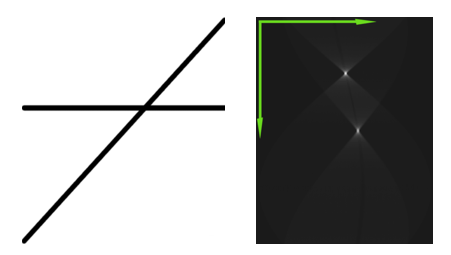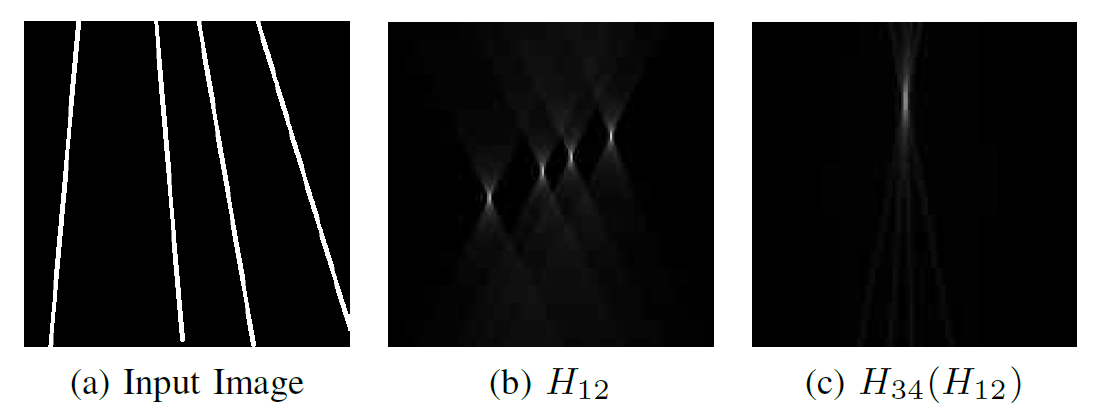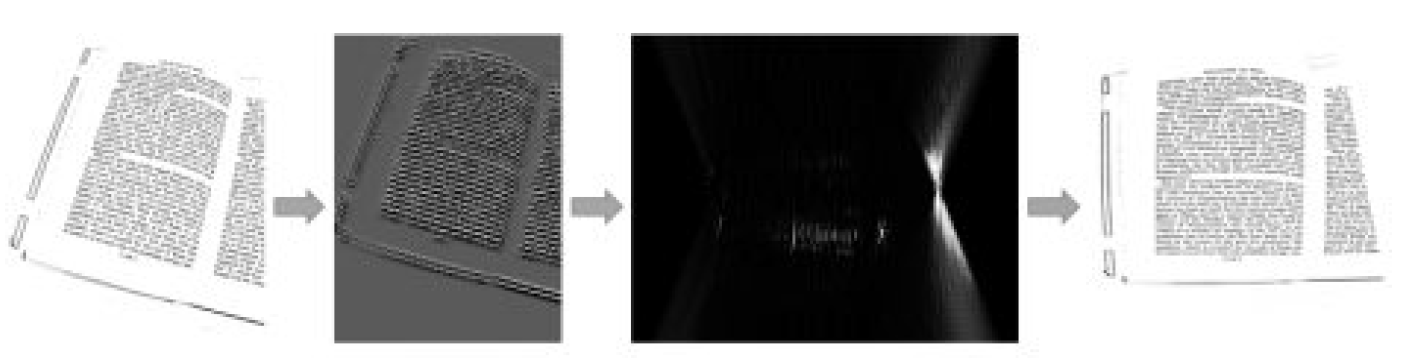# HoughNet: Search for vanishing points fused with a classic algorithm

## 7 minWhile dozens and even hundreds of proven artificial neural network (ANN) architectures are taught in the world of object recognition, warming the planet with powerful video cards and creating a “panacea” for all tasks of computer vision, we are firmly on the research path in Smart Engines, offering new effective ANN architectures to solve specific problems. Today we will talk about HafNet – a new way to search for vanishing points on images.

## Hough transformation and its quick implementation

With the development of technologies of visual systems, the list of basic algorithmic tools necessary for this has been continuously updated. So, the presence of morphological filtering, the effective calculation of convolutions, the selection of borders, and color adaptation are already considered standard for any image processing and pattern recognition framework. Increasingly, the Hough transform (discrete Radon transform) falls into the list of basic tools. The Hough transform (HX) is used to detect rectilinear objects or their various configurations in the image: detection of road markings, search for document boundaries, color segmentation, computational tomography, etc. The algorithm shows very high indices in terms of detection and is significantly more resistant to the additive coordinate and outburst noise present at the same time than, for example, the least squares method, about this, by the way, they already wrote on Habré.

Consider an arbitrary point (xi,yi) Through this point passes an infinite number of lines satisfying the equation yi= xia + b at various parameters a and b. If we rewrite this equation in the form b = -xia + yi and consider the ab plane, called the parameter space, then for a given point (xi,yi) on the original image, we obtain the equation of a single line in the parameter space. The most important property is as follows: if three points lie on one straight line in the image, then the corresponding three lines in the parameter space intersect at one point, and the coordinates of this point uniquely determine the parameters of the same straight line on which the points in the original image lay. There is a drawback in the presented parametrization: if the desired straight line is close to the vertical, then its angular coefficient tends to infinity. Therefore, in practice, other options are used to parameterize the lines (if interested, write in the comments, we will prepare a separate article about it).

Thus, the result of the Hough transform from the image is another image, the size of which is determined by the permissible values ​​of the parameters of the lines, and the pixel value is the sum of the intensities of the pixels on the corresponding line in the original image.

If there were lines in the original image, then there will be pronounced local maxima on the Hough transform. The coordinates of these maxima determine the parameters of the corresponding lines in the original image. The following figure shows the results of the Hough transform for an image with two intersecting lines.Despite such high practical value, the Hough transform has one critical drawback that often hinders the application of the Hough transform in practice: the classical implementation of the Hough transform has high computational complexity – O(n3) where n – the linear size of the investigated image.

But in practice, they often use the so-called fast Hough transform (BPH) – an algorithm that calculates the approximate value of the Hough transform, using the dyadic pattern (DP) to approximate the lines in the image. BPH complexity is rated as O(n2log (n)), which is much more pleasant from a computational point of view. At least as much as a detailed description of the BPH algorithm is beyond the scope of this article, it can be found, for example, in a scientific paper . Here, we note only an important feature of the BPH algorithm, which will be needed later in the presentation of the proposed neural network architecture: the BPH algorithm defines four quadrants of lines: “mostly vertical with a negative shift” (we will denote H1), “Mostly vertical with a positive shift” (we will denote H2), “Mainly horizontal with a negative shift” (we will denote H3) and “mostly horizontal with a positive shift” (we will denote H4) In addition, we denote H12 and H34 predominantly vertical and predominantly horizontal, respectively, obtained by combining the corresponding quadrants.

## BPH vanishing point detection

Now we are ready to deal with the principle of detection of vanishing points on the image using the Hough transform (or rather, using the Hough fast transform algorithm). Recall that the vanishing point is the point at which parallel lines of the scene in question converge on the perspective image. The set of corresponding lines is called a bundle.

Depending on the specifics of the scene, there may be a different number of vanishing points. So, for example, when we photograph a document, there are usually two vanishing points on the image: the first due to the presence of horizontal lines (both real and imaginary, such as the upper and lower borders of the document), and the second due to vertical lines. The consecutive two-time use of FHP allows you to find these vanishing points, which can then be used to correct the perspective. Let us illustrate this with an example. Consider a bunch of four lines that intersect somewhere far beyond the image. If to apply BPH to this image, then on the image H12 we will see four local maxima, each of which corresponds to a straight line in the original image. Now, if you reapply BPH, then the image H34 will contain only one local maximum, by the coordinates of which it is possible to uniquely determine the coordinates of the initial vanishing point. By the way, an interesting detail is that the sum over all the lines in the picture H12 is the same, and therefore, it does not make sense to consider the Hough transform along horizontal lines. Therefore, it is necessary to suppress the noise (in this case, we have increased the image intensity H12 squared).Similar reasoning is used to search for the second vanishing point (formed by horizontal lines of the document). Having both vanishing points, you can already correct the perspective of the image and get an even document at the output (because an even document is the key to high-quality recognition).

The curious reader Habr, who read the article before this paragraph, probably already has only one thought ripening: so where are the neural networks? It seems that the algorithm described above is extremely understandable and does not require any machine learning … So, in the next section, we are ready to give an answer to this key question!

## HoughNet Artificial Neural Locator Network

All the salt of the vanishing point search algorithm described above is that it can only be successfully used on images where the corresponding lines are somehow equally segmented (for example, as shown above, the lines are set by white pixels and the rest are black). But such an “ideal” picture of the world is extremely rare (and in truth, it never occurs at all). Therefore, before invoking the Hough transform, “correct” filtering is always applied. Only how to choose this “correct” filtering, if practically nothing is known about the scene that is fixed on the image?

After thinking, we developed the architecture of the neural network (we called it HoughNet), in which there are two layers that calculate the half-image and three groups of convolutional layers. In this combination, convolutional layers solve the problems of preliminary filtering, localizing local maxima and calculating additional statistics, and the Hough transform is a coordinate transformation that allows the neural network to work not with local pixel intensities, but with integral statistics along straight-line objects. By the way, the way to train such a neural network is also not trivial (for important details, contact )

Layer number Layer type Options Activation function
1 conv 12 filters 5×5, stride 1×1, no padding relu
2 conv 12 filters 5×5, stride 2×2, no padding relu
3 conv 12 filters 5×5, stride 1×1, no padding relu
4 Fht H12 for vertical, H34 for horizontal
5 conv 12 filters 3×9, stride 1×1, no padding relu
6 conv 12 filters 3×5, stride 1×1, no padding relu
7 conv 12 filters 3×9, stride 1×1, no padding relu
eight conv 12 filters 3×5, stride 1×1, no padding relu
nine Fht H34 for both branchesg
10 conv 16 filters 5×5, stride 3×3, no padding relu
eleven conv 16 filters 5×5, stride 3×3, no padding relu
12 conv 1 filter 5×5, stride 3×3, no padding 1 – rbf

The presented neural network generates two images: one for searching for a “vertical” vanishing point, and the second for searching for a “horizontal” vanishing point. In each image, the point with the maximum brightness value is the corresponding answer.

Let’s move on to the experiments. We trained our mesh on the open MIDV-500 dataset . This dataset contains images of various documents photographed on mobile devices. In total, 50 types of documents are presented in the dataset. A little more than half of the dataset (namely, the first 30 types of documents) was used for training, the remainder was used to assess the quality of the trained network.

Despite the fact that we trained on photographs of documents, we used the trained network as a preliminary recognition stage on the ICDAR 2011 dewarping contest dataset (which contains 100 black and white images of documents obtained by various methods and containing various geometric distortions) and measured the quality of full-text recognition.It turned out to surpass in quality not only “raw” recognition (recognition of an uncorrected image), but also the current state-of-the-art  and .

Source image Algorithm  Algorithm  Houghnet
Recognition quality 31.3% 49.6% 50.1% 59.7%